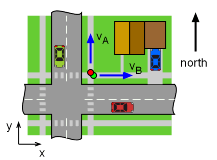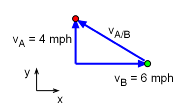Ch 1. Particle General Motion Multimedia Engineering Dynamics Position,Vel & Accel. Accel. varyw/ Time Accel. Constant Rect. Coordinates Norm/Tang. Coordinates Polar Coordinates RelativeMotion
 Chapter - Particle - 1. General Motion 2. Force & Accel. 3. Energy 4. Momentum - Rigid Body - 5. General Motion 6. Force & Accel. 7. Energy 8. Momentum 9. 3-D Motion 10. Vibrations Appendix Basic Math Units Basic Equations Sections Search eBooks Dynamics Fluids Math Mechanics Statics Thermodynamics Author(s): Kurt Gramoll ©Kurt GramollDYNAMICS - EXAMPLEJoggers Direction Example Two joggers, A and B, meet at a street corner and start jogging in different directions. Jogger A goes north at 4 mph and joggers b goes east at 6 mph. After 3 seconds, what is the relative velocity of jogger A with respect to jogger B? SolutionRelative Velocity Vector Location does not matter, relative velocity will be the same at t = 0 or t = 3 secs.      vA = vA/B + vB      4j = vA/B + 6i vA/B = -6i + 4j mph

Practice Homework and Test problems now available in the 'Eng Dynamics' mobile app
Includes over 400 problems with complete detailed solutions.
Available now at the Google Play Store and Apple App Store.